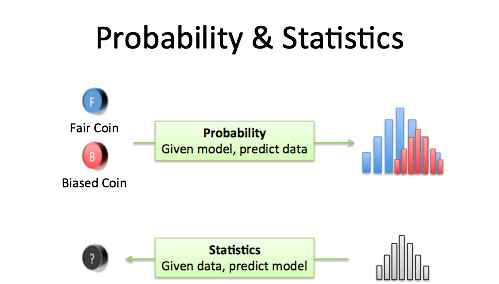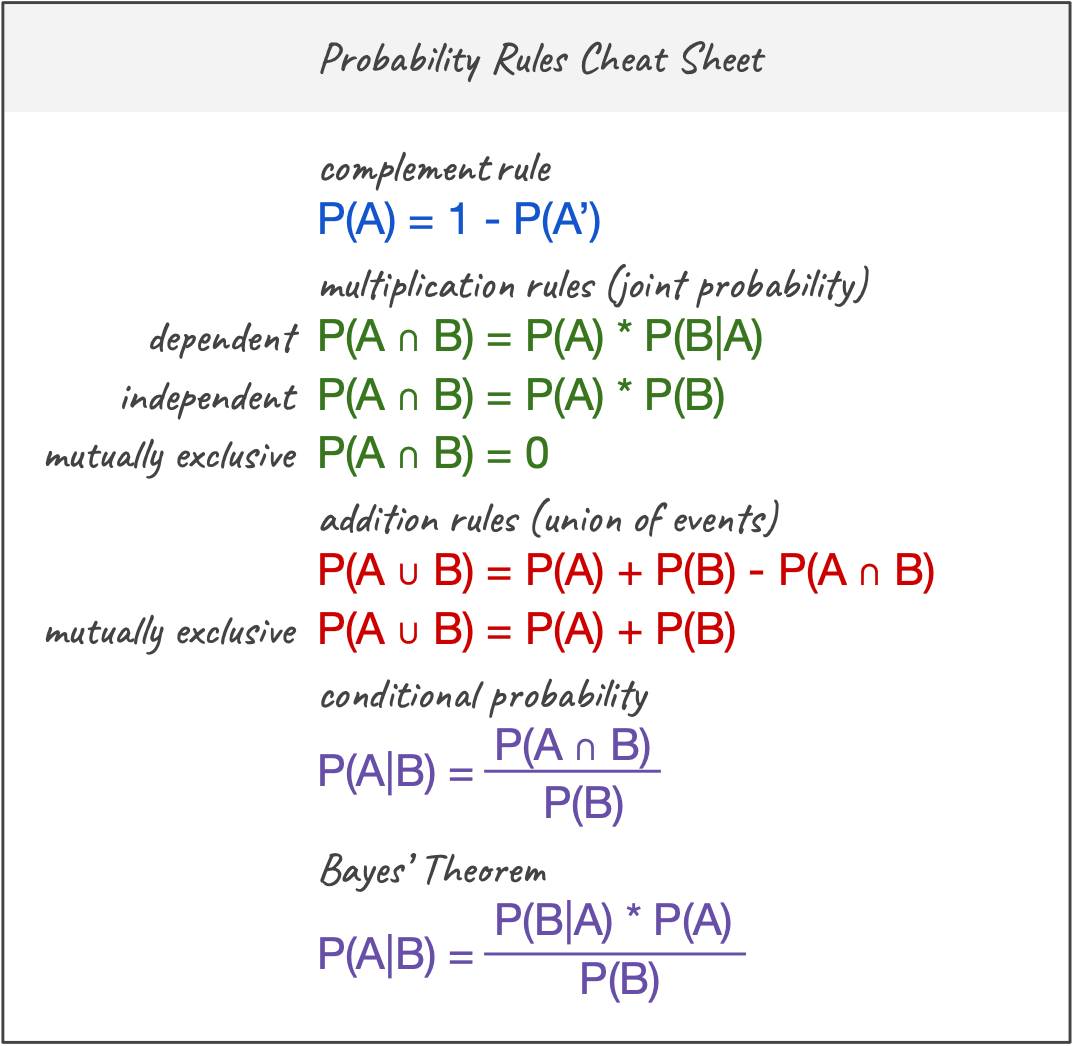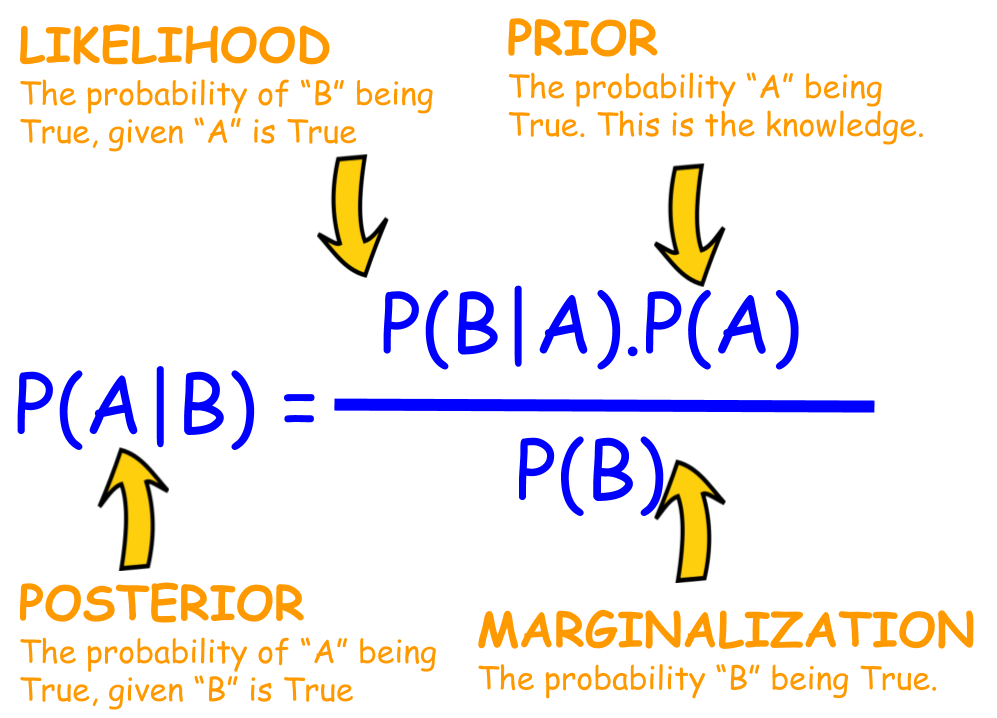# Conditional Probability## Probability vs. StatisticsProbability is used to predict the likelihood of a future event.

vs

Statistics are used to analyze past events

## Basics of ProbabilityProbability, in simple terms, is the likelihood of a situation happening. When unsure of the outcome, the probability can be calculated to know its chances.

Probability(Event)=(Number of favourable outcomes of an event) / (Total Number of possible outcomes)

The most simple example of this is a coin toss:

A coin toss can have one of 2 outcomes, either heads or tails, and both have an equal chance of happening. So the probability of either situation happening is 0.5.

The probability of certain outcomes occurring can be uneven as well.

For example is the probability of getting a prime number between 1 and 10.

In 1 to 10, there are 2,3,5,7, i.e., 4 out of 10

Hence the probability becomes 4/10 = 0.4

In 1 to 10, there is one unique number 1, i.e., 1/10

Hence probability becomes 1/10 or 0.1

In 1 to 10, there are5 composite numbers, i.e., 4,6,8,9,10

Hence the probability becomes 5/10= 0.5

Properties of probability:

1. The probability of an event can only be between 0 and 1 and can also be written as a percentage.
2. The probability of event A is often written as P(A).
3. If P(A) > P(B), then event A has a higher chance of occurring than event B.
4. If P(A) = P(B), then events A and B are equally likely to occur.
5. The Sum of probabilities of all possible situation outcomes is always 1.
6. The probability of a sure situation is 1
7. The probability of an impossible event is 0

Continuing with the above example, it is seen that

p(prime)= 0.4

p(unique)=0.1

p(composite)=0.5

1. All probabilities are between 0 and 1
2. p(composite)> p(prime) > p(unique)

Shows that selecting a composite has a higher chance of occurring than selecting a prime or unique number.

1. p(composite)=p(non-composite)=0.5 hence both are equally likely to occur.
2. Sum of probabilities

p(prime) + p(composite) + p(unique) = 0.4 + 0.1 + 0.5

= 1

If A and B are two independent events, then the probability that both will occur is equal to the product of their probabilities.

p(A ⋂ B)= p(A)* p(B)

## Conditional ProbabilityConditional probability is defined as the likelihood of an event or outcome occurring based on the occurrence of a previous event or outcome.

This is mathematically denoted by p(A/B), which means the probability of A given B has already occurred.

p(A/B) = p(A ⋂ B)/ p(B)

70% of your friends like Chocolate, and 35% like Chocolate AND like Strawberries.

What percent of those who like Chocolate also like strawberries?

P(Strawberry|Chocolate) = P(Chocolate and Strawberry) / P(Chocolate)

0.35 / 0.7 = 50%

## Bayes theorem

Bayes’s Theorem provides a principled way of calculating conditional probability.An example of a use-case for the Bayes theorem

The probability of a woman getting cancer in a region is 0.05 The machine used to test this has an 85% chance of being correct, and a woman without cancer has a 92.5% chance of getting a negative result. If a woman comes in to get tested and it comes out positive. What is the probability that she has cancer?

P(Cancer) = 0.005

P(Test Positive | Cancer) = 0.85

P(Test Neg|No cancer) = 0.925

Using the Bayes theorem:

P(Cancer|Test Positive)= P(Cancer) * P(Test Positive | Cancer) / P(Test Positive)

For this P(Test Positive) is still required

Let’s create a probability table for the same

 Probability of having cancer or not Test being positive Test being negative cancer 0.05 0.05*0.85=0.00425 0.005*0.15=0.00074 No cancer 0.995 0.995*0.075=0.074625 0.995*0.925=0.920375 Sum 1 0.078875 0.921125

### This is one of the cases when the Bayes theorem is used in real life.

Conditional probability is a fundamental concept in probability theory that measures the likelihood of an event occurring given that another event has already occurred or is known to be true. It allows us to quantify the relationship between events in situations where additional information or conditions are present.

The conditional probability of an event A given an event B is denoted as P(A|B) and is defined as the probability of event A occurring, given that event B has already occurred. It is calculated using the formula:

P(A|B) = P(A ∩ B) / P(B)

where P(A ∩ B) represents the probability of both events A and B occurring simultaneously, and P(B) is the probability of event B occurring.

Conditional probability provides a way to update our knowledge or beliefs about the occurrence of an event based on new information or conditions. It allows us to make more informed predictions and decisions by taking into account relevant context.

For example, let’s consider rolling a fair six-sided die. The probability of rolling a 5 is 1/6 since there is one favorable outcome (rolling a 5) out of six equally likely outcomes (rolling numbers 1 to 6).

Now, suppose we are given the additional information that the rolled number is odd. We want to find the probability of rolling a 5 given that the number rolled is odd. In this case, the condition is that the number is odd.

Out of the six possible outcomes, three are odd numbers (1, 3, and 5). Since we know that the number rolled is odd, we can narrow down the sample space to only the odd numbers. Therefore, the probability of rolling a 5 given that the number rolled is odd is 1/3.

Conditional probability allows us to update our probabilities based on new evidence or conditions. It is widely used in various fields such as statistics, machine learning, finance, and genetics to analyze and model complex relationships between events or variables.

Conditional probability is particularly important in Bayesian statistics, which provides a framework for incorporating prior beliefs and new evidence to update probabilities. Bayes’ theorem, a fundamental result in Bayesian statistics, allows us to calculate the conditional probability of an event given new information.

### Bayes’ theorem states:

P(A|B) = (P(B|A) * P(A)) / P(B)

where P(A) and P(B) are the probabilities of events A and B occurring independently, and P(B|A) is the conditional probability of event B given event A.

Bayes’ theorem provides a powerful tool for updating probabilities based on new evidence. It is widely used in applications such as medical diagnosis, spam filtering, and pattern recognition.

Understanding conditional probability is essential for interpreting statistical analyses, making informed decisions, and developing accurate predictive models. It allows us to reason about events in a probabilistic framework, taking into account additional information or conditions that influence the likelihood of an event.

In conclusion, conditional probability is a fundamental concept in probability theory that measures the likelihood of an event occurring given that another event has already occurred or is known to be true. It provides a way to update probabilities based on new evidence or conditions. Conditional probability is widely used in various fields and is a key component of Bayesian statistics. Understanding conditional probability is essential for probabilistic reasoning, statistical analysis, and decision-making.